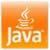# How to format Decimal Number in Java - DecimalFormat Example

We often need to format decimal numbers in Java like formatting numbers up to 2 decimal places or 3 decimal places or we want to introduce leading zeros in front of numbers. Thankfully Java programming language provides many different ways to format numbers in Java like either using Math.round() or setScale() from BigDecimal but the caveat is that they also do rounding of numbers i.e. 1.6 will be rounded on 2.0 if we use Match.round(). If we are just interested in formatting decimal numbers up to n decimal digits then DecimalFormat is the way to go. java.text.DecimalFormat descends from NumberFormat and provides a dynamic way of formatting numbers in Java.

While creating an instance of DecimalFormat you can pass a String pattern that describes on which format decimal number should be formatted and then DecimalFormat.format() method will do the rest for you. In this Java tutorial we will see how to format a decimal number in 2 decimal places, format up to 3 decimal places, using a comma to separated 3 digits, etc.

## Java DecimalFormat ExampleDecimalFormat in Java is defined in java.text package and it's a subclass of NumberFormat. In order to format a decimal number in Java, we need an instance of DecimalFormat with a predefined pattern. Once you have an instance of DecimalFormat, you can call DecimalFormat.format() method for converting any double or long number into a needed format.

Here is a code example of creating DecimalFormat and formatting numbers in Java:

import java.text.DecimalFormat;

public class DecimalFormatExample {

public static void main(String args[])  {

//formatting numbers upto 2 decimal places in Java
DecimalFormat df = new DecimalFormat("#,###,##0.00");
System.out.println(df.format(364565.14));
System.out.println(df.format(364565.1454));

//formatting numbers upto 3 decimal places in Java
df = new DecimalFormat("#,###,##0.000");
System.out.println(df.format(364565.14));
System.out.println(df.format(364565.1454));
}

}

Output:
364,565.14
364,565.15
364,565.140
364,565.145

If you look at the output of decimal format example you will see that when we used format up to 2 decimal numbers any floating-point number that has more than 2 digits after decimal point numbers will be printed only up to two digits. The same is true in the case of formatting numbers up to 3 decimal digits. if the decimal number doesn't contain 3 digits then zero will be per pended.

## Careful while Formatting Decimal numbers using DecimalFormat

Though java.text.DecimalFormat is a nice utility class and allows you to dynamically format numbers in Java it has one problem that it's not thread-safe or synchronized properly. So never share decimal format between multiple threads. It's also not advisable to cache DecimalFormat as a static resource without proper synchronization.

That's all on how to format a decimal number in Java using DecimalFormat. There are other ways also like I said but DecimalFormat is a neat, clean, and simple way of formatting numbers up to n number of decimal places.

Other Java Tutorials you may like

Unknown said...

import javax.swing.JOptionPane;
import java.text.DecimalFormat;
public class Sample3 {
public static void main(String args[]){
double amount,iRate,monPay,totalPay;
int years;
String amountStr;
String irateStr;
//takes input for loan amount,rate and loan period
amountStr = JOptionPane.showInputDialog(null,"Enter loan amount \$ : ");
irateStr = JOptionPane.showInputDialog(null,"Enter % Annual Interest: ");
yearsStr = JOptionPane.showInputDialog(null,"Enter the loan Period; ");

amount = Double.parseDouble(amountStr); //convert input String into double
iRate = Double.parseDouble(irateStr); //convert input string into double
years = Integer.parseInt(yearsStr); //convert input string into integer

monPay = (amount * iRate / 1200) /
( 1 - Math.pow( 1 / ( 1 + iRate / 1200), 12 * years)) ;//calculate monthly payment
totalPay = monPay * 12 * years;

DecimalFormat df = new DecimalFormat("0.00");
System.out.println(df.format(monPay));

JOptionPane.showMessageDialog(null,"your monthly payment is: " + monPay + "\n" +
"your total payment is: " + totalPay );
}

}

Anonymous said...

@omi tewary .In which format you want your ouput.